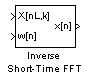# Inverse Short-Time FFT

Recover time-domain signals by performing inverse short-time, fast Fourier transform (FFT)

## Library

Transforms

`dspxfrm3`

•## Description

The Inverse Short-Time FFT block reconstructs the time-domain signal from the frequency-domain output of the Short-Time FFT block using a two-step process. First, the block performs the overlap add algorithm shown below.

`$x\left[n\right]=\frac{L}{W\left(0\right)}\sum _{p=-\infty }^{\infty }\left[\frac{1}{N}\sum _{k=0}^{N-1}X\left[pL,k\right]{e}^{j2\pi kn/N}\right]$`

Then, the block rebuffers the signal in order to reconstruct the time-domain signal. Depending on the analysis window used by the Short-Time FFT block, the Inverse Short-Time FFT block might or might not achieve perfect reconstruction of the time domain signal.

Connect your complex-valued, single-channel or multichannel input signal to the X(n,k) port. The block accepts unoriented vector, column vector and matrix input. The block outputs the real or complex-valued, single-channel or multichannel inverse short-time FFT at port x(n).

Connect your complex-valued, single-channel analysis window to the w(n) port. When you select the Assert if analysis window does not support perfect signal reconstruction check box, the block displays an error when the input signal cannot be perfectly reconstructed. The block uses the values you enter for the Analysis window length (W) and Reconstruction error tolerance, or maximum amount of allowable error in the reconstruction process, to determine if the signal can be perfectly reconstructed.

## Examples

The `dspstsa` example illustrates how to use the Short-Time FFT and Inverse Short-Time FFT blocks to remove the background noise from a speech signal. To open the `dspstsa` model, type `dspstsa` in the MATLAB® command prompt.

## Parameters

Analysis window length

Enter the length of the analysis window. This parameter is visible when you select the Assert if analysis window does not support perfect signal reconstruction check box.

Overlap between consecutive STFFT frames (in samples)

Enter the number of samples of overlap for each frame of the Short-Time FFT block's input signal. This value should be the same as the Overlap between consecutive windows (in samples) parameter in the Short-Time FFT block parameters dialog.

Samples per output frame

Enter the desired frame size of the output signal.

Input is conjugate symmetric

Select this check box when the input to the block is both floating point and conjugate symmetric, and you want real-valued outputs. When you select this check box when the input is not conjugate symmetric, the output of the block is invalid. This parameter cannot be used for fixed-point signals.

Assert if analysis window does not support perfect signal reconstruction

Select this check box to display an error when the analysis window used by the Short-Time FFT block does not support perfect signal reconstruction.

Reconstruction error tolerance

Enter the amount of acceptable error in the reconstruction of the original signal. This parameter is visible when you select the Assert if analysis window does not support perfect signal reconstruction check box.

## Supported Data Types

PortSupported Data Types

X(n,k)

• Double-precision floating point

• Single-precision floating point

w(n)

• Double-precision floating point

• Single-precision floating point

x(n)

• Double-precision floating point

• Single-precision floating point

 Quatieri, Thomas E. Discrete-Time Speech Signal Processing. Englewood Cliffs, NJ: Prentice-Hall, 2001.

Watch now